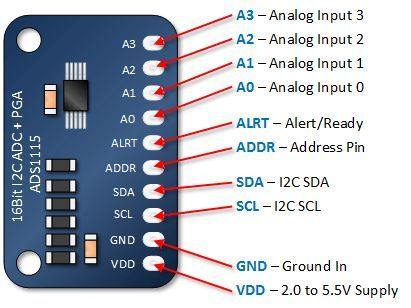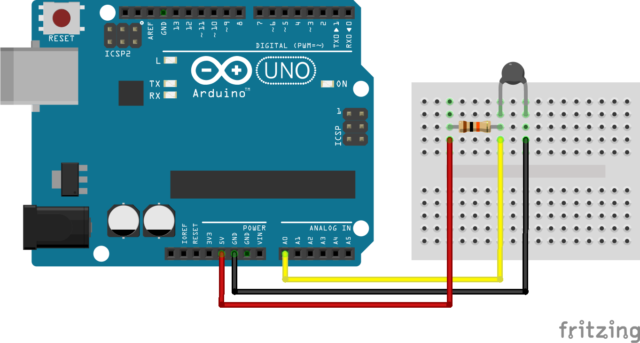# Universal temp sensor sketch works with resistance devices (NTC, PTXXX) and thermocouples (typeK and probably others)

hi there here a sketch for a universal temperature sensor usage resistance or thermocouples one needs to use ads1115 as it is 16 bit for it to work universally.
it works well and pretty accurate for all sensors I have tried NTC, PT and type K.
For resistance sensors, set up sensor connection as follows with the appropriate resistor i.e. 10K NTC a 10 k resistor – PT1000 a 1 k resistor etc …Hook up the the thermo resistors the same way you would on an arduino but using the analog ports of ads1115 insteadFor thermocouples you can leave out the resistor and red wire connection as you are only using ground and analog connection. the blue wire of the thermocouple to ground and red wire of thermocouple to the analog port.

How it works for thermocouples is I take the mV read of the sensor and convert it in to pseudo resistance (i.e. 0mV = 1K - 1mv = 0.9k, and 1mV = 1.1K). When calibrating the thermocouple you mark down the pseudo resistance at a particular temperature. You need 3 of them. The greater the range between them the better. Then add those temps and resistance in this Thermistor Calculator

``````#include <math.h>
float Vo = 3.29; /// accurate measure of your base voltage
int sample = 50;
float To = 298.15; // Nominal Temperature

// β coefficients of  resistance
float Ro1 = 10, B =  3950; //Nominal resistance 50K, Beta constant
float R1 = 10;// Series resistor 10K

float Ro2 = 1.10201, B2 = -316.44; // //kilo ohms
float R2 = 1;  //kilo ohms

float Ro3 = 10, B3 =  3950; //Nominal resistance 50K, Beta constant
float R3 = 10;// Series resistor 10K

float Ro4 = 10, B4 =  3950; //Nominal resistance 50K, Beta constant
float R4 = 10;// Series resistor 10K

// Steinhart-Hart coefficients  for resistance and  thermocouplers

int TYPE_1 =0;  // 0 for resister type 1 for thermo coupler
const double A1 = 0.0010059783701056113;   /// example 10k 3950
const double b1 = 0.0002564639419274676;
const double C1 = -1.596587342625349e-8;

int TYPE_2 =0;   // 0 for resister type 1 for thermo coupler
const double A2 = 0.06501312861989986;     /// example PT1000
const double b2 = -0.01161716353567784;
const double C2 = 0.00005732815001133613;

int TYPE_3 =1;    // 0 for resister type 1 for thermo coupler
const double A3 = 0.0137109609005272;       /// example type K
const double b3 = -0.0018058420165089223;
const double C3 = 0.000006228131786341517;

int TYPE_4 = 0;    // 0 for resister type 1 for thermo coupler
const double A4 = 0.0010059783701056113;
const double b4 = 0.0002564639419274676;
const double C4 = -1.596587342625349e-8;

float Tc1 =0; // NTC 10
float Tc2 =0;  // PT1000
float Tc3 =0;  // type K
float Tc4 =0;   // NTC 10
double Tc2a =0;
double Tc2b =0;
double Tc2c =0;
double Tc2d =0;

float Via=0;
float Vib=0;
float Vic=0;
float Vid=0;

float ohm1 =0;
float ohm2 =0;
float ohm3 =0;
float ohm4=0;

float Vi = 0;
//float f2 =0;

void setup() {
Serial.begin(115200);
Serial.println(__FILE__);

}

void loop()
{
THERMO();

Serial.print("β coefficients #1 "); Serial.println( Tc1, 3 );
Serial.print("β coefficients #2 ");Serial.println( Tc2, 3 );
Serial.print("β coefficients #3 ");Serial.println( Tc3, 3 );
Serial.print("β coefficients #4 ");Serial.println( Tc4, 3 );
Serial.println("");
Serial.print("Steinhart-Hart coefficients  for #1 "); Serial.println(Tc2a ,3);
Serial.print("Steinhart-Hart coefficients  for #2 "); Serial.println(Tc2b ,3);
Serial.print("Steinhart-Hart coefficients  for #3 ");Serial.println(Tc2c ,3);
Serial.print("Steinhart-Hart coefficients  for #4 "); Serial.println(Tc2d ,3);

//Serial.print("K ohm on #2 "); Serial.println(ohm2, 6);
Serial.println("------------------------- ");
delay(100);
Tc1 = 0; // resets thermo sampling
Tc2 = 0;
Tc3 = 0;
Tc4 = 0;
Tc2a = 0;
Tc2b = 0;
Tc2c = 0;
Tc2d = 0;
ohm1 = 0;
ohm2 = 0;
ohm3 = 0;
ohm4 = 0;
Via = 0;
Vib = 0;
Vic = 0;
Vid = 0;

}

void THERMO() {
float T1 = 0;
float T2 = 0;
float T3 = 0;
float T4 = 0;

for (int i = 0; i <= sample; i++) {

delay(50);

float f = ADS.toVoltage(1);  // voltage factor
float f1=(val_0 * f);
float f2=(val_1 * f);
float f3=(val_2 * f);
float f4=(val_3 * f);
// Serial.println(f1, 4); //prints the voltage of  f1

Vi = f1;

//All Resistance are in kilohms.
float R = (Vi * R1 ) / (Vo - Vi);
float T =  1 / ((1 / To) + ((log(R / Ro1)) / B));
float TcA = T - 273.15; // Converting kelvin to celsius
T1 = (1 / (A1 + (b1 * log(R*1000)) +  (C1 * ( log(R*1000) * log(R*1000) * log(R*1000))))-273.15 );
Via =Via +Vi;
Tc1 = Tc1+TcA;
Tc2a = Tc2a+T1;
ohm1 = ohm1 + R;
/////////////
Vi = f2;
R = (Vi * R2 ) / (Vo - Vi);

T =  1 / ((1 / To) + ((log(R /Ro2)) / B2));
float TcB = T - 273.15; // Converting kelvin to celsius
Tc2 = Tc2+TcB;
T2 = (1 / (A2 + (b2 * log(R*1000)) +  (C2 * ( log(R*1000) * log(R*1000) * log(R*1000))))-273.15 );
ohm2 = ohm2 + R;
Vib =Vib +Vi;
Tc2b = Tc2b+T2;
//////////////
Vi = f3;
R = (Vi * R3 ) / (Vo - Vi);
T =  1 / ((1 / To) + ((log(R / Ro3)) / B3));
float TcC = T - 273.15; // Converting kelvin to celsius
T3 = (1 / (A3 + (b3 * log(R*1000)) +  (C3 * ( log(R*1000) * log(R*1000) * log(R*1000))))-273.15 );
Vic =Vic +Vi;
Tc2c = Tc2c+T3;
Tc3 = Tc3+TcC;
ohm3 = ohm3 +R;
Vi = f4;
R = (Vi * (R4 )) / (Vo - Vi);
T =  1 / ((1 / To) + ((log(R / Ro4)) / B4));
float TcD = T - 273.15; // Converting kelvin to celsius
T4 = (1 / (A4 + (b4 * log(R*1000)) +  (C4 * ( log(R*1000) * log(R*1000) * log(R*1000))))-273.15 );
Vid =Vid +Vi;
Tc4 = Tc4+TcD;
Tc2d = Tc2d+T4;
ohm4 =ohm4+ R;
}

ohm1=ohm1/sample;
ohm2=ohm2/sample;
ohm3=ohm3/sample;
ohm4=ohm4/sample;

Serial.println("");
Serial.print( "max volt"); Serial.println( ADS.getMaxVoltage() );
Serial.print("Kohm on #1 "); Serial.println(ohm1, 5);
Serial.print("Kohm on #2 "); Serial.println(ohm2, 5);
Serial.print("Kohm on #3 "); Serial.println(ohm3, 5);
Serial.print("Kohm on #4 "); Serial.println(ohm4, 5);
Tc1 = Tc1/sample;
Tc2 = Tc2/sample;
Tc3 = Tc3/sample;
Tc4 = Tc4/sample;
Tc2a=Tc2a/sample;
Tc2b=Tc2b/sample;
Tc2c=Tc2c/sample;
Tc2d=Tc2d/sample;

if (TYPE_1 == 1){
float P_R = (ohm1 *100) +1;
Tc2a = (1 / (A1 + (b1 * log(P_R*1000)) +  (C1 * ( log(P_R*1000) * log(P_R*1000) * log(P_R*1000))))-273.15 );

Serial.print("pseudo Kohm on #1 "); Serial.println(P_R, 5);
}
if (TYPE_2 == 1){
float P_R = (ohm2 *100) +1;
Tc2b = (1 / (A2 + (b2 * log(P_R*1000)) +  (C2 * ( log(P_R*1000) * log(P_R*1000) * log(P_R*1000))))-273.15 );

Serial.print("pseudo Kohm on #2 "); Serial.println(P_R, 5);
}
if (TYPE_3 == 1){
float P_R = (ohm3 *100) +1;
Tc2c = (1 / (A3 + (b3 * log(P_R*1000)) +  (C3 * ( log(P_R*1000) * log(P_R*1000) * log(P_R*1000))))-273.15 );
Serial.print("pseudo Kohm on #3 "); Serial.println(P_R, 5);
}
if (TYPE_4 ==1){
float P_R = (ohm4 *100) +1;
Tc2d = (1 / (A4 + (b4 * log(P_R*1000)) +  (C4 * ( log(P_R*1000) * log(P_R*1000) * log(P_R*1000))))-273.15 );

Serial.print("pseudo Kohm on #4 "); Serial.println(P_R, 5);
}
// Serial.print("volt ") ; Serial.print(Via, 8); Serial.print(" ");
Serial.println("");
return;
}
// -- END OF FILE --
``````

and as you can see they are fairly close I used the PT1000 one for determine the temperature of the medium the type k sensor was in

and similar at 0C

This is a pretty easy and cheap method. A K-type sensor reader for Arduino is \$15.
The same for PT. An ads1115 is \$5 and you can do 4 sensors at once compared to only 1 with the type K break out.

Universaltemperturesensor.zip (2.1 KB)

if you find your NTC temp stops reading temperature around 15C change the gain on the ADS1115
ADS.setGain(1); works well for NTC but the temps will vary a bit for PT and type K
ADS.setGain(2); works best for PT and type k ( NTC works well too but only if above 15C
ADS.setGain(3); all work but Type k will vary a bit
ADS.setGain(4); works best with type k - thermo couplers ( resistance do not work )

Here’s an image of how it is wired up. I used terminal blocks for the resistors so I can insert different resistors for different sensors or leave them out for thermocouples